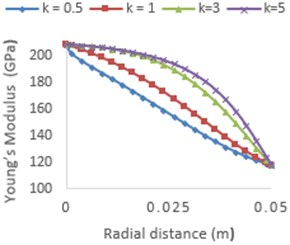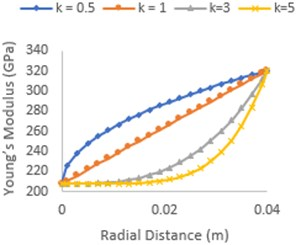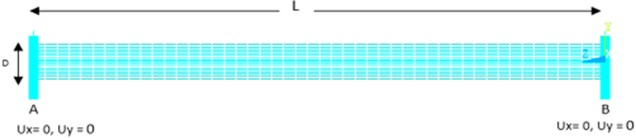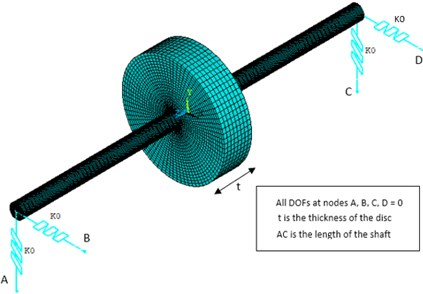# Natural frequency analysis of a functionally graded rotor system using three-dimensional finite element method

## Arnab Bose1, Prabhakar Sathujoda2

1, 2Bennett University, Greater Noida, India

1Corresponding author

Vibroengineering PROCEDIA, Vol. 29, 2019, p. 70-75. https://doi.org/10.21595/vp.2019.21099
Received 14 October 2019; accepted 21 October 2019; published 28 November 2019

43rd International Conference on Vibroengineering in Greater Noida (Delhi), India, November 28-30, 2019

Copyright © 2019 Arnab Bose, et al. This is an open access article distributed under the Creative Commons Attribution License, which permits unrestricted use, distribution, and reproduction in any medium, provided the original work is properly cited.
Views 375
Abstract.

Three-dimensional Finite Element (FE) analysis has been carried out using ANSYS software to study the natural frequencies of functionally graded (FG) rotor system. Temperature and position-dependent material properties of the FG shaft system are considered to be graded in the radial direction. Power-law with the nonlinear temperature distribution (NLTD) and exponential law with exponential temperature distribution (ETD) have been used to model the material gradation and temperature distribution. Rotor systems of two different FG materials, namely Stainless Steel-ZrO2 and Stainless Steel-Al2O3 have been studied. Python codes have been developed to generate ANSYS macros that apply the material properties. Simply supported FG shaft and FG rotor system supported on bearings have been analyzed in the current work. The results show the influence of different power-law coefficients, different material and material laws on the natural frequencies of the rotor system. The effect of these parameters on whirl frequencies has also been studied for FG rotor systems.

Keywords: functionally graded shaft, finite element, whirl frequency.

#### 1. Introduction

A typical functionally graded material (FGM), with a high bending–stretching coupling effect, is an inhomogeneous composite made from different phases of material constituents (usually ceramic and metal). The volume fraction of the constituent materials is gradually varied; thus, the material properties exhibit a smooth and continuous change from one surface to another. Ceramic constituents of FGMs can withstand high-temperature environments due to their better thermal resistance characteristics, while the metal constituents provide stronger mechanical performance and reduce the possibility of fracture. Rotor dynamics analysis of FG rotor forms the base of the current work.

Some of the important works in this direction are presented in the following paragraphs. Nelson and McVaugh , presented a procedure for dynamic modeling of rotor-bearing systems which consist of rigid disks and discrete bearings. The formulation was presented in both a fixed and rotating frame of reference. Zori and Nelson  study extends the linear finite element concept to provide a detailed evaluation of damped rotor stability. Prabhakar et al  worked on crack detection and vibration characteristics of cracked shafts . The works reported in References [1-3] dealt with the dynamic analysis of rotors made of homozeneous isotropic materials.

The first practical application of FGMs was carried out at the National Aerospace Laboratory of Japan in 1984 to prepare advanced ultra-high temperature resistance structural materials . An elasticity solution was provided for FG beams by Sankar , using exponential law. Reddy and Chin , studied the dynamic thermoelastic response of functionally graded cylinders and plates using finite element modeling and considering the effect of thermomechanical coupling in the formulation. Gayen and Roy  work deal with the study of vibration and stability analysis of a functionally graded spinning shaft system using beam elements based on the Timoshenko beam theory.

Most of the previous works in FG systems has been performed using beam elements. As FGM are finding widespread use in industries, analysis using three-dimensional elements for accurate modeling of the problems is necessary. Thus, the present work aims to study the vibration analysis of a shaft and a rotor system, modeled using ANSYS three-dimensional hex elements by considering two different FG materials and temperature distribution laws.

#### 2. Material modelling

To accurately model the properties of FGMs, the properties should be temperature and position dependent. In the case of circular shafts, the radial distribution of properties is present. The temperature-dependent material properties P can be written as in  shown in Eq. (1):

(1)
$P\left(T\right)={P}_{0}\left({P}_{-1}{T}^{-1}+1+{P}_{1}{T}^{1}+{P}_{2}{T}^{2}+{P}_{3}{T}^{3}\right),$

where ${P}_{0}$,${P}_{-1}$, ${P}_{1}$, ${P}_{2}$, ${P}_{3}$are the coefficients of temperature $T$ (in K) and are unique to the constituent materials. Typical values of these constants for material properties like Young’s modulus (in Pa), Poisson’s ratio, thermal expansion coefficient (in K-1), and the thermal conductivity (in W mK-1) of ceramics and metals can be obtained from the works of Reddy and Chin 1998 . For a hollow cylinder having temperatures ${T}_{o}$ and ${T}_{i}$ on outer and inner surface respectively, temperature distribution at any radial distance, $r$ is given by Eq. (2):

(2)
$\frac{d}{dr}\left[rK\left(r\right)\frac{dT}{dr}\right]=0.$

The position-dependent material properties $P\left(r\right)$ for a circular FG shaft or cylinder can be expressed by using Eq. (3), . $Pm$ and $Pc$ represents material properties at ceramic rich and metal-rich region respectively as a function of temperature. $Ro$ and $Ri$ are the outer and inner radii of the shaft, respectively. $k$ is the power-law index. NLTD is the solution of Eq. (2), and considering the first seven terms of the polynomial expansion , yields Eq. (4). Where $Kcm=Kc–Km$. The density at each layer has been given by Eq. (5):

(3)
(4)
$T\left(r\right)=Tm+\left(Tc-Tm\right)\left[\sum _{j=0}^{5}\left\{\frac{{\left(-1\right)}^{j}}{jk+1}{\left(\frac{Kcm}{Km}\right)}^{j}{\left(\frac{r-Ri}{Ro-Ri}\right)}^{jk+1}\right\}\right]/\left[\sum _{j=0}^{5}\frac{{\left(-1\right)}^{j}}{jk+1}{\left(\frac{Kcm}{Km}\right)}^{j}\right]$
(5)

#### 3. Code validation

Python code has been developed to generate ANSYS material macros, which can be used to apply the position dependent material properties to a rotor shaft system. To verify the material properties obtained using the developed code, a solid FG shaft composed of Stainless Steel (SS) and Zirconia (ZrO2) having outer diameter ($Ro$) 100 mm, a ceramic rich region at a temperature of 900 K and metal core at a temperature of 300 K has been considered. The material properties have been calculated at different radial distances, and a graph is plotted for different power-law coefficients ($k$) as shown in Fig. 1. Material properties are validated by the works of Gayen et al. . After validation, the code is extended to different a FG material composed of Al2O3 and SUS304 for 80 mm diameter shaft at room temperature. Radial variation of Young’s Modulus from Python output is in good agreement with works of Gayen and Roy , which is shown in Fig. 2. Thus, the code is adopted for further works in different FG shaft systems discussed in current work. The points in the plots show the value plotted which lies exactly above the curves obtained from [7, 10].

Fig. 1. Young’s modulus variation (SS-ZrO2)Fig. 2. Young’s modulus variation (SS-Al2O3)#### 4. FE modeling and solution procedure

In the present work, two separate three-dimensional FE models are developed in ANSYS software. The first model is a simply supported FG shaft without disc which is used to validate the FE model with the available literature results. Whereas the second model is an FG shaft supported on bearings and containing a centrally located steel disc. 3-Dimensional 8 noded structural SOLID185 elements having three degrees of freedom per node, have been considered for modeling shaft and disc. The simply supported condition is achieved by fixing displacements in non-axial directions on all nodes present on end faces as shown in Fig. 3. The simply supported FE model is further used to determine the natural frequencies of FG shafts having different material gradations in present work.

Fig. 3. Meshed model of simply supported FG ShaftFig. 4. Meshed FE model of rotor bearing systemBesides SOLID185 elements, COMBIN 14 elements are used to model the bearings in the second model. COMBIN 14 element takes the bearing stiffness and damping as an input. The displacements at the end nodes of these elements are fixed as shown in Fig. 4 The rotor-bearing model shown in Fig. 4, is used for validation work and has been further used for obtaining the whirl frequencies under different material gradation.

Block Lanczos eigenvalue extraction method is used for obtaining natural frequencies of the simply supported shaft. The whirl frequency extraction of the rotor-bearing system has been carried out using QR damped solver of ANSYS.

#### 5. Results and discussions

A modal analysis of two FE models is performed in ANSYS software to obtain the natural frequencies and mode shapes. Before presenting the results of rotor systems having different material gradations, the developed three-dimensional ANSYS FE models are validated by comparing the modal analysis results (namely the natural frequencies) with works from literature. The validation results are given in the following Sections of 5.1 and 5.2 and further results are presented in Section 5.3 and 5.4.

#### 5.1. A nonrotating simply supported FG shaft – 3D FE model validation

The first second natural frequencies are obtained for nonrotating simply supported FG shaft composed of SUS304 and Al2O3 with power-law coefficient $k=$ 0.5 and $L/D$ ratio as 12.5. The radius of the shaft is taken as 0.04 m and the corresponding length is 1 m. The obtained natural frequencies have been compared with works of Gayen et al. , on an uncracked shaft of the same dimension and material. The temperature has been taken as room temperature and the results showed significant agreement as shown in Table 1.

#### 5.2. A steel rotor-bearing system – 3D FE model validation

A rotor bearing system made of steel (as shown in Fig. 4) consisting of a rotor, a central disc and bearings is considered for the validation of the 3D FE model. The radius and length of the solid shaft are 0.01 m and 0.5 m respectively. The disc is located at midspan and is 0.04 m thick and has a radius of 0.075 m. The density and elastic modulus of steel rotor system is 7800 kg/m3 and 2·08 E11 N/m2 respectively. The bearings stiffness and damping coefficients are taken as 10E5 N/m and 100 Ns/m. The first two natural frequencies are obtained from ANSYS modal analysis, and they show significant confirmation with works of Sekhar and Prasad , as listed in Table 2.

Table 1. Natural frequency validation

 Modes Present Gayen  Difference (%) Simply supported FG (steel/Al2O3) shaft, $k=$ 0.5 First 255.99 255.92 0.027 Second 1003.5 1017.71 1.4

Table 2. Natural frequency validation

 Modes Present Sekhar and Prasad  Difference (%) Steel rotor-bearing system First 24.2 24.27 0.28 Second 94.5 95.2 0.73

#### 5.3. Analysis of simply supported FG shaft using different parameters

Further work is carried out to study the natural frequency analysis of FG shafts (shown in Fig. 3) composed of SS-Al2O3 and SS-ZrO2 at a constant temperature of 300 K using power-law material gradation. Different power-law coefficients have been used. The results obtained from ANSYS modal analysis are tabulated in Table 3. The natural frequencies show a decreasing trend with the increase in power-law coefficients for both the materials. Also, at the same power-law coefficients, natural frequencies of SS-Al2O3 shaft are higher compared to SS-ZrO2 shaft. This is expected as SS-Al2O3 FG shaft has higher stiffness, which can be seen from the graphs in Fig. 1 and Fig. 2.

Table 3. Natural frequencies of simply supported FG shafts using different parameters

 Modes FGM comprising (steel and Al2O3) FGM comprising (steel and ZrO2) Power law Power law $k$ $k$ 0.5 1 3 5 0.5 1 3 5 1st 255.9 239.2 209.3 197.2 221.9 203.2 178.8 171.7 2nd 1003.5 937.3 819.8 772.1 870.8 797.7 701.8 673.8

#### 5.4. Analysis of rotor-bearing system

The rotor-bearing system validated in Section 5.2 has been used to investigate effect of different material gradation on the natural frequencies. Two different FG materials are considered in the present work as discussed in Section 5.3. The dimensions of rotor system, stiffness and damping constants of the bearings are kept the same as in Section 5.2. An isothermal temperature of 300 K is considered in the analysis. The natural frequencies of different FG rotor models from ANSYS modal analysis is tabulated in Table 4, for different material laws, FG materials and for different power-law coefficients. The natural frequencies show similar trend as observed in Section 5.3. The decrease in natural frequencies with increasing $k$ values is due to decrease in stiffness of the rotor. Therefore, in FG shafts, power-law gradation $k$ influences the natural frequency and hence k may be chosen to keep the overall stiffness of the shaft and the temperature resistance within desired limit. The whirl frequencies of the rotor-bearing systems have also been analyzed at different rotation speeds. The first two forward whirl (FW) frequencies are shown in Table 5. Significant backward whirl frequencies (BW) have not been obtained as internal damping effects of the shaft has not been considered. BW frequencies decrease with the increase in $k$. The frequencies decrease due to decrease in stiffness with increasing power law coefficients. Therefore, in order to keep the whirl frequencies of an FG shaft within a desired limit power-law index $k$ can be chosen appropriately.

Table 4. Natural frequencies of rotor bearing FG shaft systems using different parameters

 Modes FGM comprising (steel and Al2O3) FGM comprising (steel and ZrO2) Power law Power law $k$ $k$ 0.5 1 3 5 0.5 1 3 5 1st 26.62 26.41 25.94 25.71 25.14 24.99 24.71 24.61 2nd 112.5 108.9 102.4 100.0 118.7 112.8 103.0 99.56 3rd 459.0 432.3 383.18 363.3 420.0 385.4 339.7 326.3

Table 5. Whirl frequencies of rotor-bearing systems using different parameters

 Operation speeds(rad/s) Modes FGM comprising (steel and Al2O3) FGM comprising (steel and ZrO2) 0.5 0.5 10 1FW 26.608 25.145 2FW 105.933 111.782 1000 1FW 26.608 25.145 2FW 176.975 202.485

#### 6. Conclusions

Three-dimensional FE modelling and analysis of the FG rotor systems has been carried out using ANSYS to study the influence of FG material gradation on the natural frequencies. ANSYS macro files are generated using python code which can be read to achieve the layered gradation of material properties.

Power law and NLTD has been used for material gradation and the temperature distribution respectively. Power law coefficients ($k$) have been varied and a decrease in natural frequencies with increasing k values has been observed. Two different FG materials are studied and SS-ZrO2 shows lower natural frequency at a fixed $k$ value. The whirl frequencies have also been studied, since no significant internal damping, backward whirl has not been observed. Further work is planned on the dynamic analysis of FG rotor system using different FG materials and gradation laws, effect of internal damping and crack inclusion in the shafts.

1. Nelson H. D., Mcvaugh J. M. The dynamics of rotor bearing systems using finite elements. Journal of Manufacturing Science and Engineering, Vol. 98, 1976, p. 593-600. [Publisher]
2. Zorzi E. S., Nelson H. D. Finite element simulation of rotor-bearing systems with internal damping. Journal of Engineering Gas Turbines Power, Vol. 99, Issue 1, 1977, p. 71-76. [Publisher]
3. Prabhakar S., Sekhar A. S., Mohanty A. R. Detection and monitoring of cracks using mechanical impedance of rotor bearing system. Journal of Acoustical Society of America, Vol. 110, 2001, p. 2351-2359. [Publisher]
4. Gayen D., Tiwari R., Chakraborty D. Static and dynamic analyses of cracked functionally graded structural components: a review. Composites Part B: Engineering, Vol. 173, 2019, p. 106982. [Publisher]
5. Sankar B. V. An elasticity solution for functionally graded beams. Composites Science and Technology, Vol. 61, 2001, p. 689-696. [Publisher]
6. Reddy J. N., Chin C. D. Thermoelastical analysis of functionally graded cylinders and plates. Journal of Thermal Stresses, Vol. 21, 2008, p. 593-626. [Publisher]
7. Gayen D., Chakraborty D., Tiwari R. Whirl frequencies and critical speeds of a rotor-bearing system with a cracked functionally graded shaft – finite element analysis. European Journal of Mechanics/A Solids, Vol. 61, 2017, p. 47-58. [Publisher]
8. Shen H. S. Functionally Graded Materials Nonlinear Analysis of Plates and Shells. CRC, Florid, 2009. [Search CrossRef]
9. Lanhe W. Thermal buckling of a simply supported moderately thick rectangular FGM plate. Composite Structures, Vol. 64, Issue 2, 2004, p. 211-218. [Publisher]
10. Gayen D., Roy T. Finite element based vibration analysis of functionally graded spinning shaft system. Journal of Mechanical Engineering Science: Part C, Vol. 228, Issue 18, 2014, p. 3306-3321. [Publisher]
11. Gayen, D., Chakraborty D., Tiwari R. Free vibration analysis of functionally graded shaft system with a surface crack. Journal of Vibration Engineering and Technologies, Vol. 6, 2018, p. 483-494. [Publisher]
12. Sekhar A. S., Prasad P. B. Dynamic analysis of a rotor system considering a slant crack in the shaft. Journal of Sound and Vibration, Vol. 208, Issue 3, 1997, p. 457-474. [Publisher]

#### Cited By

 Mathematical Problems in Engineering Vijayakumar Vaka, Prabhakar Sathujoda, Neelanchali Asija Bhalla, Y. V. Satish Kumar, Roberto Citarella, Chiara Bedon 2022 Applied Sciences Prabhakar Sathujoda, Aneesh Batchu, Giacomo Canale, Roberto Citarella 2022 Forces in Mechanics Vijayakumar Vaka, Prabhakar Sathujoda, Neelanchali Asija Bhalla, Y V Satish Kumar 2022 MATERIALS, MECHANICS & MODELING (NCMMM-2020) Arnab Bose, Prabhakar Sathujoda 2021 Journal of Structural Integrity and Maintenance Prabhakar Sathujoda, Aneesh Batchu, Bharath Obalareddy 2021 Materials Prabhakar Sathujoda, Bharath Obalareddy, Aneesh Batchu, Giacomo Canale, Angelo Maligno, Roberto Citarella 2020 Applied Sciences Prabhakar Sathujoda, Aneesh Batchu, Bharath Obalareddy, Giacomo Canale, Angelo Maligno, Roberto Citarella 2020